## Exercise 1

Determine the sides of the largest isosceles triangle that can fit within a circle with a radius of 12 cm.

## Exercise 2

An isosceles triangle with a perimeter of 30 cm turns about the vertical axis generating a three dimensional cone. What should the length of the base be in order to maximize the volume of the resultant cone?

## Exercise 3

A manufacturer seeks to produce a cylindrical can (complete with a lid) with a capacity of one liter. What should the dimensions of the can be in order to minimize the amount of metal used?

## Exercise 4

Decompose the number 44 into two summands such that five times the square of the first summand over six times the square of the second summand is the minimum possible resultant.

## Exercise 5

A 1 m long peice of wire needs to be divided into two pieces which will form the shape of a circle and a square. Determine the length of each piece so that the sum of the areas of the circle and square is minimized.

## Exercise 6

Find the dimensions of the largest possible rectangle that can be drawn in an isosceles triangle whose base is 10 cm and height, 15 cm.

## Exercise 7

The surface area cost of construction per for a large box is \$50 for the base, \$60 for the top and \$40 for the walls. Find the dimensions of this box that minimize the cost if its volume has to be 9 and its height, 1 m.

## Exercise 8

Cutting squares out of each corner of a sheet of cardboard creates a box without a lid. If the cardboard sheet has dimensions of 80 cm x 50 cm, determine the size of each equally sized square to be removed from the cardboard sheets (see figure) in order to maximize the volume of the resultant box.## Solution of exercise 1

Determine the sides of the largest isosceles triangle that can fit within a circle with a radius of 12 cm.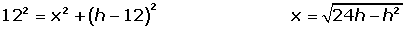## Solution of exercise 2

An isosceles triangle with a perimeter of 30 cm turns about the vertical axis generating a three dimensional cone. What should the length of the base be in order to maximize the volume of the resultant cone?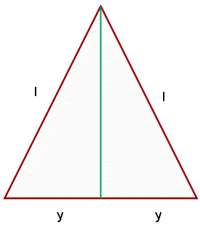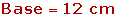## Solution of exercise 3

A manufacturer seeks to produce a cylindrical can (complete with a lid) with a capacity of one liter. What should the dimensions of the can be in order to minimize the amount of metal used?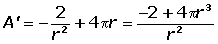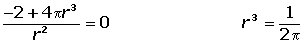## Solution of exercise 4

Decompose the number 44 into two summands such that five times the square of the first summand over six times the square of the second summand is the minimum possible resultant.## Solution of exercise 5

A 1 m long peice of wire needs to be divided into two pieces which will form the shape of a circle and a square. Determine the length of each piece so that the sum of the areas of the circle and square is minimized.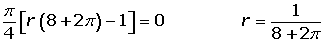## Solution of exercise 6

Find the dimensions of the largest possible rectangle that can be drawn in an isosceles triangle whose base is 10 cm and height, 15 cm.By having two similar triangles it's true that: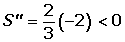## Solution of exercise 7

The surface area cost of construction per for a large box is \$50 for the base, \$60 for the top and \$40 for the walls. Find the dimensions of this box that minimize the cost if its volume has to be 9 and its height, 1 m.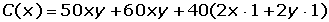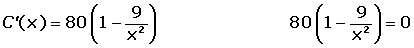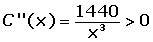## Solution of exercise 8

Cutting squares out of each corner of a sheet of cardboard creates a box without a lid. If the cardboard sheet has dimensions of 80 cm x 50 cm, determine the size of each equally sized square to be removed from the cardboard sheets (see figure) in order to maximize the volume of the resultant box.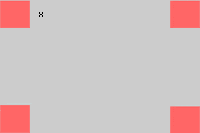Did you like the article?(No Ratings Yet)Loading...

Emma

I am passionate about travelling and currently live and work in Paris. I like to spend my time reading, gardening, running, learning languages and exploring new places.

Did you like
this resource?

Bravo!Download it in pdf format by simply entering your e-mail!

{{ downloadEmailSaved }}

Your email is not valid